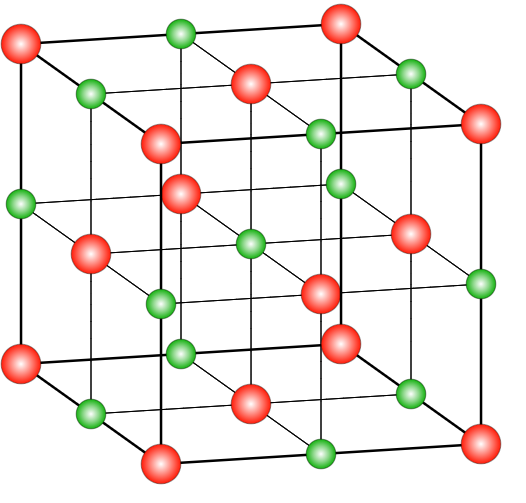QuestionAnswers

# The crystalline structure of $NaCl$ isA. Hexagonal close packingB. Face-centred cubic C. square planarD. body-centred cubicHint: A unit cell that has a lattice point at every face centre in addition to the lattice point at every corner is called a face-centred cubic unit cell. In the case of $NaCl$, the ratio of chloride to the ratio of sodium ions is 1:1.

In the case of $NaCl$, the structure is a rock salt type structure with coordination number being in a ratio of 6:6 which means that there are 6 chloride ions present on the 6 faces of the face centred cubic system and 6 Na+ ions occupy the octahedral voids in the lattice system. There are many compounds that have a similar structure as the sodium chloride structure. The ratio of radius range of anions and cations ranges between 225-414 nm. Those structures which have a similar structure to that of $NaCl$ include different alkyl halides, oxides of different metals, etc. In the case of a face-centred cubic system, the coordination number will be 12 and the distance between the two nearest atoms will be $\dfrac{{a\sqrt 2 }}{2}$ . The relation between the radius and side length in such a system is $r = \frac{a}{{2\sqrt 2 }}$ .
Thus, the correct option is (B) Face-centred cubic.where red spheres represent $Cl^-$ and green ones are for $Na^+$

Note:
In a face centred cubic system, along the face diagonal, all the atoms touch each other and the length of the face diagonal is $a\sqrt 2$ . The number of atoms per unit cell in FCC lattice is 4 and the packing efficiency is 74% with void space of 26%.
View Notes
Structure of AtomStructure of Acetylene - The Triple BondsAtomic StructureThe Solid StateThe Difference Between an Animal that is A Regulator and One that is A ConformerWhat is the full form of phd?Chemical Bonding and Molecular StructureWhere There is a Will There is a Way EssayThe Making of a ScientistChanging the Period of a PendulumImportant Questions for CBSE Class 12 Chemistry Chapter 1 - The Solid StateNCERT Books Free Download for Class 12 Chemistry Chapter 1 - The Solid StateImportant Questions for CBSE Class 11 Chemistry Chapter 2 - Structure of AtomImportant Questions for CBSE Class 9 Science Chapter 4 - Structure of The AtomImportant Questions for CBSE Class 12 Chemistry Chapter 7 - The p-Block ElementsImportant Questions for CBSE Class 12 Chemistry Chapter 8 - The d and f Block ElementsImportant Questions for CBSE Class 6 English A Pact with The Sun Chapter 8 - A Pact with the SunImportant Questions for CBSE Class 12 ChemistryImportant Questions for CBSE Class 12 Chemistry Chapter 5 - Surface ChemistryImportant Questions for CBSE Class 6 English A Pact with The Sun Chapter 1 - A Tale of Two BirdsChemistry Question Paper for CBSE Class 12CBSE Class 12 Chemistry Question Paper 2020Chemistry Question Paper for CBSE Class 12 - 2013Chemistry Question Paper for CBSE Class 12 - 2015CBSE Class 12 Chemistry Question Paper 2019 - Free PDFCBSE Class 12 Chemistry Question Paper 2017 - Free PDFCBSE Class 12 Chemistry Question Paper 2018 - Free PDFPrevious Year Question Paper for CBSE Class 12 Chemistry - 2014Chemistry Question Paper for CBSE Class 12 - 2016 Set 1 EChemistry Question Paper for CBSE Class 12 - 2016 Set 1 SLakhmir Singh Chemistry Class 9 Solutions Chapter 4 - Structure Of The AtomNCERT Solutions for Class 12 Chemistry Chapter 1NCERT Solutions for Class 12 Chemistry Chapter 1 The Solid State in HindiNCERT Solutions for Class 11 chemistry chapter 2 – Structure of AtomNCERT Solutions for Class 11 Chemistry Chapter 2 Structure of Atom In HindiNCERT Solutions for Class 9 Science Chapter 4 Structure of The Atom in HindiNCERT Solutions for Class 11 Chemistry Chapter 4NCERT Exemplar for Class 9 Science Chapter 4 - Structure of The Atom (Book Solutions)NCERT Solutions for Class 9 Science Chapter 4Surface Chemistry NCERT Solutions - Class 12 Chemistry